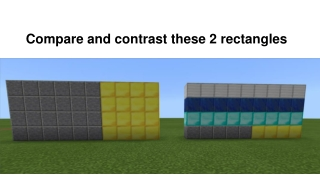DownloadDownload PresentationCompare and contrast these 2 rectangles

# Compare and contrast these 2 rectangles

Download Presentation## Compare and contrast these 2 rectangles

- - - - - - - - - - - - - - - - - - - - - - - - - - - E N D - - - - - - - - - - - - - - - - - - - - - - - - - - -
##### Presentation Transcript

1. Compare and contrast these 2 rectangles

2. Why is this rectangle=(6x4)+(4x4)

3. Why is this rectangle=4x(6+4)

4. Compare and contrast (AxB)+(AxC) = Ax(B+C)

5. What is the area of the floor space? Don’t include walls or door spaces 6 9 9 6+9+9=24

6. What do these math models have in common (AxB)+(AxC)

7. What is the perimeter of this home? 8+8+5+5=26

8. To build the walls just stack up loops of the perimeter, use glass loops for windows. DON’T COUNT THE FLOOR. How many blocks are in the walls? 26+26+26+26=64

9. The Big Idea: Math is needed to create buildings and measuring the area and perimeter of shapes are its tools.